# Minimizing the Cost of Borrowing Stablecoins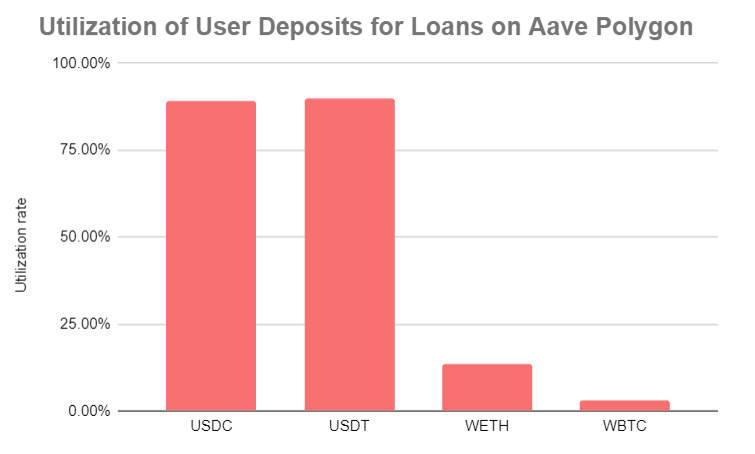The graph above shows the percentage of deposits for each collateral type that is lent out.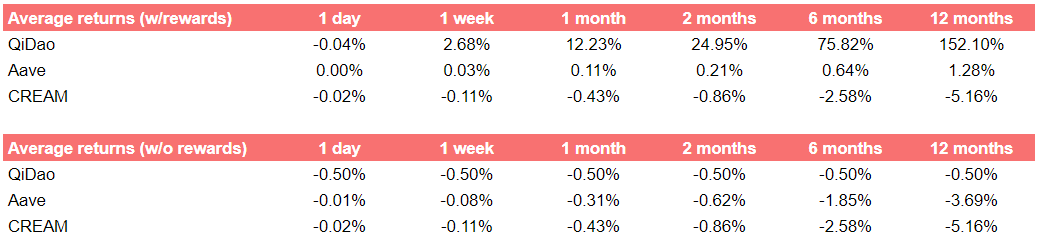Note: negative numbers reflect a cost; positive numbers reflect a gain.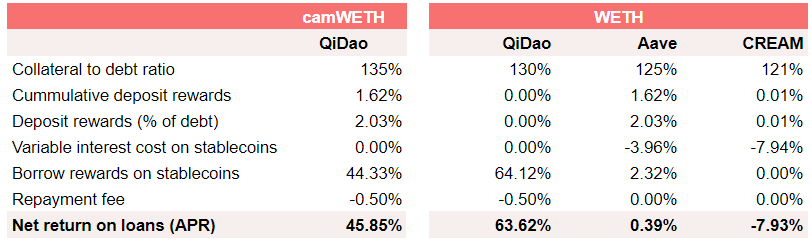Note: negative numbers reflect a cost; positive numbers reflect a gain.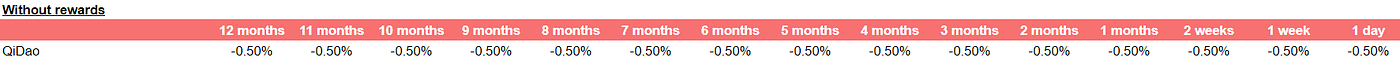Note: negative numbers reflect a cost; positive numbers reflect a gain.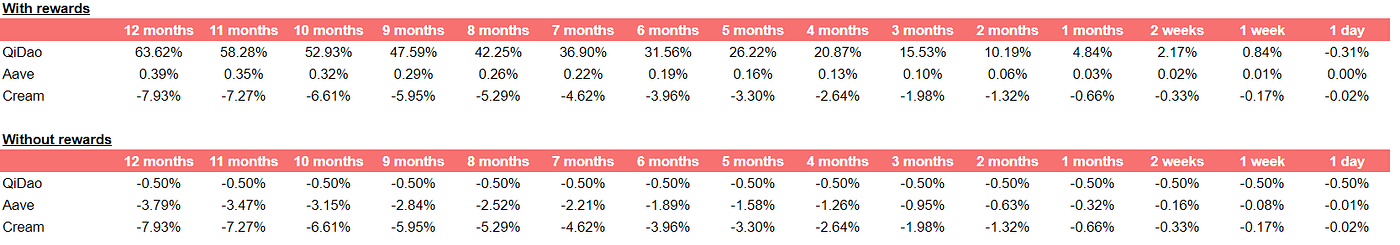Note: negative numbers reflect a cost; positive numbers reflect a gain.

--

--